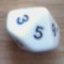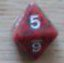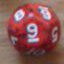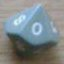Why won't this work?

## Why won't this work?

 Sat Nov 17 01:44:49 2018   by   vxi I'm getting a parse error on line 4, but I see nothing wrong with it. I'm sorry if it's obvious. Thank you for any help you can providea:=d8;w:=d20;t:=0;if a = w then t:=2; else t:=0;if a > w then t:=1; else t:=0;if a = {4,6,8} & w = {2,4,6} then t:=t+1; else t:=0;if a = {3,5,7} & w = {1,3,5} then t:=t+1; else t:=0;if t = 2 then 1 else 0 Sun Nov 18 01:45:05 2018   by   wyrdR if a = w then t:=2; else t:=0;if a > w then t:=1; else t:=0;if a = {4,6,8} & w = {2,4,6} then t:=t+1; else t:=0;if a = {3,5,7} & w = {1,3,5} then t:=t+1; else t:=0;should bet:=if a = w then 2 else 0;t=:if a > w then 1 else 0;t:=if a = {4,6,8} & w = {2,4,6} then t+1 else 0;t:=if a = {3,5,7} & w = {1,3,5} then t+1 else 0; Sun Nov 18 02:03:04 2018   by   wyrdR There's still issues, and I made a mistake. I'm testing a few things out... Be back soon. Sun Nov 18 02:32:27 2018   by   wyrdR a:=d8;w:=d20;t:=0;t:= if a > w then 1 else 0;    \ if a is larger t = 1t:= if a = w then 2 else t;    \ if equal to = 2t:=if a = {4,6,8} & w = {2,4,6} then t+1 else t;      t:=if a = {3,5,7} & w = {1,3,5} then t+1 else t;    t Mon Nov 19 05:51:38 2018   by   vxi Thank you! This format makes perfect sense.